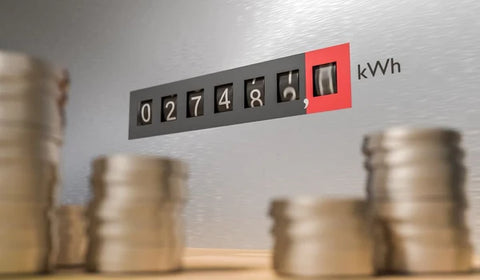Watts To Volts Calculator - ShopSolar.com

# Watts To Volts Calculator

When working with solar power, it helps to have a basic understanding of electrical terms and calculations.

One of the most useful tools is the watts-to-volts calculator. Don’t stress if you have very limited electrical know-how. We explain everything very simply, in the short guide below.

 Select current type: DC AC - Single phase AC - Three phase Enter power in watts: W Enter current in amps: A Enter power factor: Voltage result in volts: V

## Watts vs Volts vs AmpsBefore we get to how to convert watts to volts, you need to understand some basic terminology.

This will help you convert watts to volts, convert watts into amps, or convert watts to kVA (kilovolt amps - more about those in a bit).

Let’s run through a brief description of these basic electrical units.

### Watts

Watts are the basic unit of electrical power. Watts are used to describe the power used by appliances, whereas kilowatts are usually used to describe the power used in a residential or commercial setting.

How do you convert watts to kilowatts? A thousand watts gives you 1 kilowatt. Therefore, 1,200 watts is equal to 1.2 kilowatts. The prefix, kilo-, means 1,000.

Because watts are equal to amps multiplied by, they are also sometimes described as volt-amperes or volt-amps.

### Volts

Volts are the unit of measurement of electrical potential flowing through a system.

And like watts, volts can also have the prefix kilo- for readings in the thousands. Thus, 1 kilo-volt-ampere is equal to 1,000 volt-ampere.

### Amps

Amperage (or amps) is the unit that measures the flow of electrical current in a circuit. Whereas volts represent the amount of energy with which electrons pass a specific point within a closed circuit, amps measure the volume of electrons present in the circuit.

## Watts to Volts CalculatorNow that you have the basic terminology covered, it’s time to learn how to convert watts to volts. It’s very simple. Just follow the formula below.

V (Volts) = P (Watts) / I (Amps)

What this means is:

The voltage V in volts (V) is equal to the power P in watts (W), divided by the current I in amps (A). Put another way, if you know the amps you can calculate the volts.

Let’s go through an example so you can see how this formula works.

At 1 amp, a watt is always equal to a volt. Thus, if you have a 1 amp circuit, 1 volt is equal to 1 watt.

However, amps make the difference, too. So at 10 amps, 1 volt is equal to 10 watts. And if you have a 100 amp circuit, 1 volt is equal to 100 watts.

## Why Do I Need to Know All this for Installing Solar Panels?

Although solar panels harness the power of the sun’s heat and light, this power is converted to electricity for use in your home. The basic electrical principles that apply to all appliances apply here as well.

However, there are some principles unique to solar power. For example, a wattage calculator for solar panels

This calculates the theoretical power production of your solar panels. But it is reliant on external factors like the amount of direct sunlight your panels receive.

If you need a simple watt calculator for a generator, just remember that 1 watt = 1 volt × 1 ampere.

## Watts to Volts Calculator Conclusion

Installing solar panels in your home, RV, or any other situation is simple when you have the right knowledge, tools, and supplies.

You can also make use of our solar load calculator to determine your needs.

You can find all of the solar & electrical calculators we have created in order to help you plan, size and install your own solar power system here.

## Join the Solar Lab!